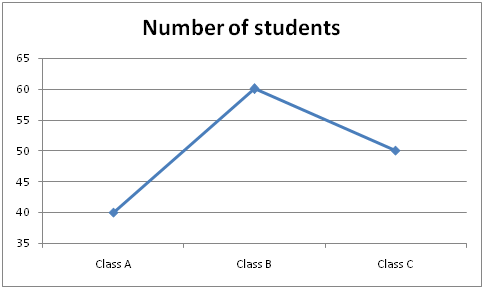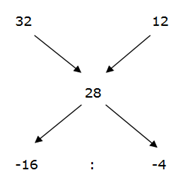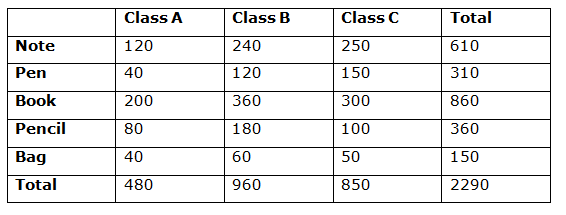# SBI Clerk Mains Quantitative Aptitude (Day-04)

Dear Aspirants, Our IBPS Guide team is providing new series of Quantitative Aptitude Questions for SBI Clerk Mains 2020 so the aspirants can practice it on a daily basis. These questions are framed by our skilled experts after understanding your needs thoroughly. Aspirants can practice these new series questions daily to familiarize with the exact exam pattern and make your preparation effective.

Start Quiz

Ensure Your Ability Before the Exam – Take SBI Clerk 2020 Mains Free Mock Test

Application Sums

1) The average age of P, Q and R, 3 years ago was 14 years and the average present age of P, Q, R and S together is 20 years. Find the present age of S?

a) 29 years

b) 32 years

c) 36 years

d) 27 years

e) None of these

2) P starts the business with the capital of Rs. 15000. Q joins the business after 4 months and R joins the business after 6 months. At the end of the year, their respective shares are in the ratio of 18: 16: 15. Find the sum of the investment of Q and R together?

a) Rs. 40000

b) Rs. 50000

c) Rs. 55000

d) Rs. 45000

e) None of these

3) The average marks obtained by 65 students in a certain examination are 28. The average marks of passed students is 32 and the average marks of failed students is 12. Then find the total number of passed students?

a) 52

b) 56

c) 48

d) 54

e) None of these

4) Akila borrowed a certain sum of money at simple interest for 4 years at 10 % per annum and he pays Rs. 20000 as interest. Find the compound interest for the same amount at 8 % per annum for 2 years?

a) Rs. 8320

b) Rs. 7860

c) Rs. 8040

d) Rs. 8580

e) None of these

5) Three pipes P, Q and R can fill a tank in 24 hours. After working together for 8 hours, R is closed and P and Q can fill the remaining part in 20 hours. How much time taken by R to fill the tank alone?

a) 100 hours

b) 85 hours

c) 120 hours

d) 105 hours

e) None of these

Data Interpretation

Directions (6 – 10): Study the following information carefully and answer the given questions?

The line graph shows the number of students in three different classes (A, B and C).A teacher distributes five different stationary items viz. Note, Pen, Book, Pencil and Bag in each class for each student.

In class A, teacher distributes 3 notes, 1 pen, 5 books, x pencil and (x – 1) bag for each student. Total item distributed in the class A is 480.

In class B, teacher distributes 4 notes, y pens, (y + 4) books, (x + 1) pencils and (y – 1) bags for each student. Total items distributed in the class B is twice the total item distributed in the class A.

In class C, total notes distributed by teacher is 10 notes more than total notes distributed in class B and the ratio of total books distributed in class A to C is 2: 3. And then distributes (y + 1) pens, x pencils and z bags for each student.

The teacher distributed a total of 2290 stationary items to all the classes.

6) Quantity I: Find the average number of items distributed in class A

Quantity II: Find the average number of items distributed in class B

a) Quantity I < Quantity II

b) Quantity I = Quantity II

c) Quantity I ≥ Quantity II

d) Quantity I ≤ Quantity II

e) Quantity I > Quantity II

7) What is the ratio of the total pencil to total bags distributed for all the schools together?

a) 7: 9

b) 8: 11

c) 12: 5

d) 6: 7

e) 5: 4

8) Total pen and pencil distributed for school B together is what percentage more/less than the total note and pen distributed in class C together?

a) 20% more

b) 20% less

c) 40% less

d) 25% less

e) 25% more

9) Which of the following stationary items distributed maximum for all the class together?

a) Pen

b) Note

c) Books

d) Pencil

e) Bag

10) What is the difference between the number of Note, pen and bags distributed for all the class together to that of the remaining items distributed for all the class together?

a) 130

b) 180

c) 190

d) 110

e) None of these

Directions (1-5) :

The average age of P, Q and R, 3 years ago = 14 years

The total present age of P, Q and R = (14 * 3) + 9 = 51 years

The average present age of P, Q, R and S together = 20 years

The total present age of P, Q, R and S together = 80 years

The present age of S = 80 – 51 = 29 years

Shortcut:

Average present age of P, Q and R = 14 + 3 = 17

The present age of S = 20 + (20 – 17) * 3 = 29 years

The share of P, Q and R,

= > [15000 * 12]: [Q * 8]: [R * 6] = 18: 16: 15

= > 180000: 8Q: 6R = 18: 16: 15

18’s = 180000

1’s = 10000

8Q = 160000

Q = 20000

6R = 150000

R = 25000

The sum of the investment of Q and R together

= > 20000 + 25000

= > Rs. 45000

Let passed students be x,

According to the question,

65 * 28 = 32 * x + 12 * (65 – x)

1820 = 32x + 780 – 12x

1040 = 20x

x = 1040 / 20 = 52

Total number of passed students = 52

(Or)= > 4 : 1

5’s = 65

1’s = 13

The total number of passed students = 52

SI = PNR / 100

20000 = (P * 4 * 10) / 100

P = (20000 * 100) / 40 = Rs. 50000

Compound Interest:

CI for 1st year = 50000 * (8/100) = 4000

CI for 2nd year = 54000 * (8/100) = 4320

CI = 4000 + 4320 = Rs. 8320

Or

CI = P (1 + r/100)n – P

= 50000 (1 + 8/100)2 – 50000

= 58320 – 50000

= Rs. 8320

The tank will be filled by P, Q, R in 8 hours = 8/24 = 1/3

Remaining work = 1 – 1/3 = 2/3

Time taken by P and Q to fill the tank = 20/2 * 3 = 30 h

R’s 1 hour work = 1/24 – 1/30 = 1/120

Time taken by R to fill the tank = 120 hrs

Directions (6 – 10):

Class A:

Total notes = 3 * 40 = 120

Total pens = 40 * 1 = 40

Total books = 40 * 5 = 200

Total pencils = 40 * x

Total bags = 40 * (x – 1)

Total item = 120 + 40 + 200 + 40x + 40 (x – 1) = 480

80x – 40 = 120

= > 80x = 160 = > x = 2

Total pencils = 40 * x = 80

Total bags = 40 * (x – 1) = 40

Class B:

Total notes = 60 * 4 = 240

Total pens = 60 * y

Total books = 60 * (y + 4)

Total pencils = 60 * (x + 1) = 60 * (2 + 1) = 180

Total bags = 60 * (y – 1)

Total items = 480 * 2 = 960

240 + 60y + 60y + 240 + 180 + 60y – 60 = 960

600 + 180y = 960

= > y = 360/180 = 2

Total pens = 60 * y = 120

Total books = 60 * (y + 4) = 360

Total bags = 60 * (y – 1) = 60

Class C:

Total notes = 240 + 10 = 250

Total books = 200/2 * 3 = 300

Total pens = 50 * (y + 1) = 50 * (2 + 1) = 150

Total pencils = 50 * x = 50x = 50 * 2 = 100

Total bags = 50 * z

Total items = 2290 – 480 – 960 = 850

250 + 300 + 150 + 100 + 50z = 850

50z = 50

= > z = 1

Total bags = 50 * 1 = 50Quantity I:

Required average = 480/40 = 12

Quantity II:

Required average = 960/60 = 16

Hence, Quantity I < Quantity II

Required ratio = 360: 150 = 12: 5

Required percentage

= [(250 + 150) – (120 + 180)]/(150 + 250) * 100

= 100/400 * 100 = 25% less

Note = 610

Pen = 310

Books = 860

Pencil = 360

Bag = 150

Total Note, pen and bags distributed for all the class together

= (610 + 310 + 150) = 1070

Remaining items = 2290 – 1070 = 1220

Required difference = 1220 – 1070 = 150

 Check Here to View SBI Clerk Mains 2020 Quantitative Aptitude Questions Day 03 Day 02 Day 01 Click Here for SBI Clerk 2020 – Detailed Exam Notification